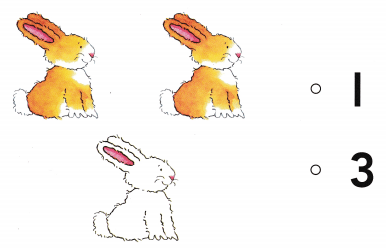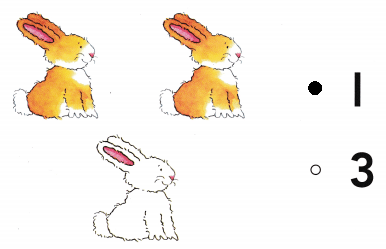# Texas Go Math Kindergarten Lesson 9.3 Answer Key Decompose Numbers Up to 5

Refer to our Texas Go Math Kindergarten Answer Key Pdf to score good marks in the exams. Test yourself by practicing the problems from Texas Go Math Kindergarten Lesson 9.3 Answer Key Decompose Numbers Up to 5.

## Texas Go Math Kindergarten Lesson 9.3 Answer Key Decompose Numbers Up to 5

Explore

DIRECTIONS: Place 5 counters in the five frame as shown. Trace the counters. Trace the number that shows how many counters are red. Trace the symbol. Write the number of counters that are yellow.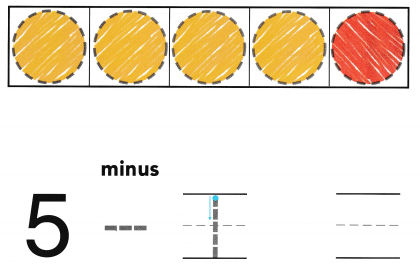Explanation:
The number of red counters are 1
the number of yellow counters are 4
Total number of counters 5
5 – 1 = 4

Share and Show

DIRECTIONS: 1. Place 4 counters in the five frame as shown. Trace the counters. Write the number of counters that are red. Write the number of counters that are yellow. Trace the symbol. 2. Place 3 counters in the five frame as shown. Trace the counters. Write the number of counters that are red. Write the number of counters that are yellow. Trace the symbol.

Question 1.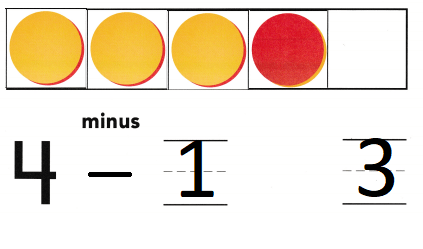Explanation:
The number of red counters are 1
the number of yellow counters are 3
Total number of counters 4
4 – 1 = 3

Question 2.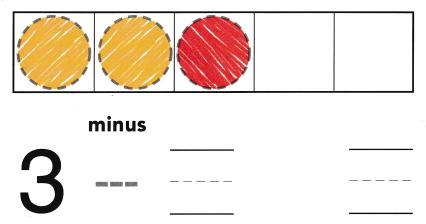Explanation:
The number of red counters are 1
the number of yellow counters are 2
Total number of counters 3
3 – 1 = 2

DIRECTIONS: 3. Place 4 counters in the five frame. Trace the counters. Write the number of counters that are red. Write the number of counters that are yellow. Trace the symbol. 4. Place 3 counters in the five frame. Trace the counters. Write the number of counters that are red. Write the number of counters that are yellow. Trace the symbol.

Question 3.Explanation:
The number of red counters are 2
the number of yellow counters are 2
Total number of counters 4
4 – 2 = 2

Question 4.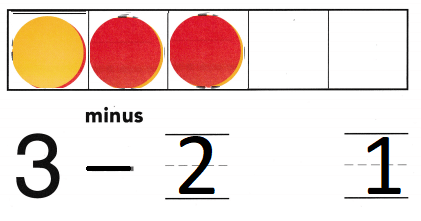Explanation:
The number of red counters are 2
the number of yellow counters are 1
Total number of counters 3
3 – 2 = 1

HOME ACTIVITY • Show your child a set of 5 small objects. Take away three objects. Have him or her describe how you decomposed the set.Explanation:
John has 5 trucks
He gave 3 to his friend
5 – 3 = 2
so, 2 trucks are remaining

DIRECTIONS: 5. There are counters in the five frame. One counter is yellow. How many counters are red? Color the counters. Write the number of counters in the five frame. Write the number of counters that are yellow. Write the number of counters that are red. 6. Choose the correct answer. There are 4 rabbits. Two of the rabbits are big. How many rabbits are small?

Problem Solving

Question 5.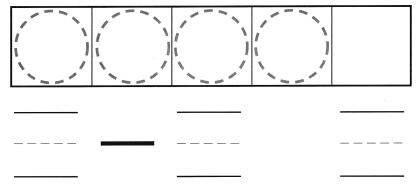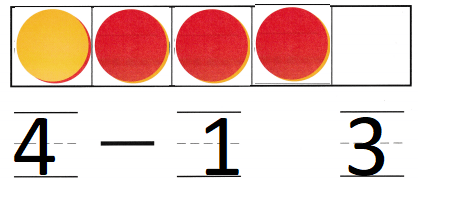Explanation:
The number of red counters are 3
the number of yellow counters are 1
Total number of counters 4
4 – 1 = 3

Question 6.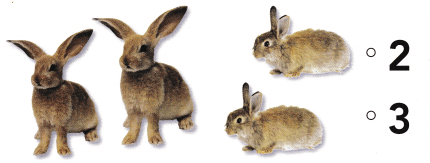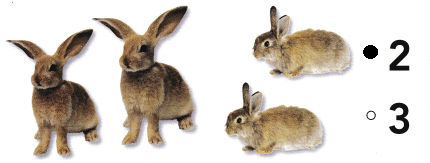Explanation:
There are 4 rabbits. Two of the rabbits are big.
4 – 2 = 2
2 rabbits are small
The difference of 4 and 2 is 2

### Texas Go Math Kindergarten Lesson 9.3 Homework and Practice Answer Key

DIRECTIONS: 1. There are 5 counters in the five frames. Trace the symbol and write the number of counters that are red. Write the number of counters that are yellow. 2. There are 4 counters in the five frame. Trace the symbol and write the number of counters that are red. Write the number of counters that are yellow.

Question 1.Explanation:
The number of red counters are 2
the number of yellow counters are 3
Total number of counters 5
5 – 2 = 3

Question 2.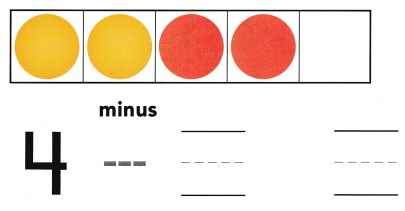Explanation:
The number of red counters are 2
the number of yellow counters are 2
Total number of counters 4
4 – 2 = 2

DIRECTIONS: Choose the correct answer. 3. There are 5 turtles. Three of the turtles are green. How many turtles are brown? 4. There are 3 rabbits. Two of the rabbits are brown. How many rabbits are white?

Lesson Check

Question 3.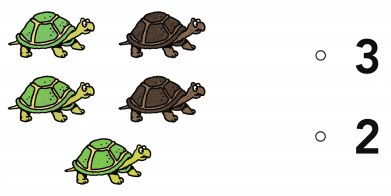Explanation:
There are 5 turtles. Three of the turtles are green.
5 – 3 = 2
2 turtles are brown

Question 4.